## RS Aggarwal Class 7 Solutions Chapter 7 Linear Equations in One Variable Ex 7A

These Solutions are part of RS Aggarwal Solutions Class 7. Here we have given RS Aggarwal Solutions Class 7 Chapter 7 Linear Equations in One Variable Ex 7A.

Other Exercises

Solve the following equations. Check your result in each case.
Question 1.
Solution:
3x – 5 = 0
3x – 5 + 5 = 0 + 5
⇒ 3x = 5
⇒ x = $$\frac { 5 }{ 3 }$$
Check:
L.H.S. = 3x – 5
= 3 x $$\frac { 5 }{ 3 }$$ – 5
= 5 – 5
= 0
= R.H.S.
Hence x = $$\frac { 5 }{ 3 }$$

Question 2.
Solution:
8x – 3 = 9 – 2x
⇒ 8x + 2x = 9 + 3 (By transposing)
⇒ 10x = 12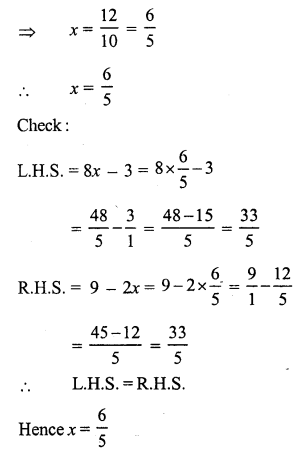Question 3.
Solution:
7 – 5x = 5 – 7x
⇒ – 5x + 7x = 5 – 7 (By transposing)
⇒ 2x = -2
x = -1
Check:
L.H.S. = 7 – 5x = 7 – 5(-1) = 7 + 5 = 12
R.H.S. = 5 – 7x = 5 – 7(-1) = 5 + 7 = 12
L.H.S. = R.H.S.
Hence x = -1

Question 4.
Solution:
3 + 2x = 1 – x
⇒ 2x + x = 1 – 3 (By transposing)
⇒ 3x = -2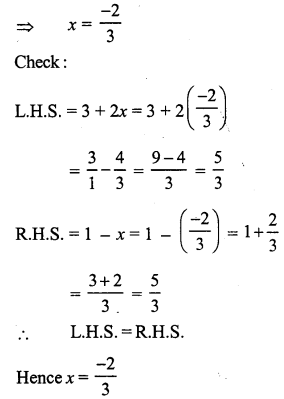Question 5.
Solution:
2(x – 2) + 3(4x – 1) = 0
⇒ 2x – 4 + 12x – 3 = 0
⇒ 2x + 12x = 4 + 3 (By transposing)
⇒ 14x = 7
⇒ x = $$\frac { 7 }{ 14 }$$ = $$\frac { 1 }{ 2 }$$
Check : L.H.S. = 2(x – 2) + 3 (4x -1)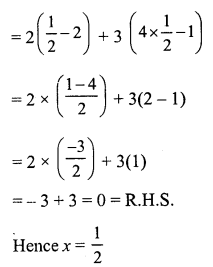Question 6.
Solution:
5 (2x – 3) – 3(3x – 7) = 5
⇒ 10x – 15 – 9x + 21 = 5
⇒ 10x – 9x – 15 + 21 = 5
⇒ 10x – 9x = 5 + 15 – 21 (By transposing)
⇒ x = 20 – 21 = -1
⇒ x = -1
Check:
L.H.S. = 5 (2x – 3) – 3(3x – 7)
= 5[2 x (-1) -3] -3[3 (-1) -7] = 5[-2 – 3] – 3[-3 – 7]
= 5 x (-5) -3 x (-10)
= -25 + 30
= 5 = R.H.S.
Hence x = -1

Question 7.
Solution: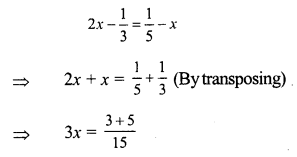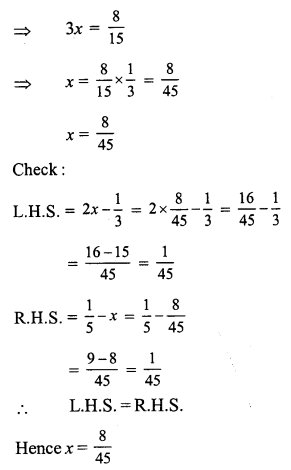Question 8.
Solution:L.H.S. = R.H.S.
Hence x = 48

Question 9.
Solution: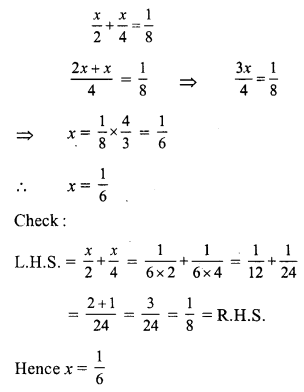Question 10.
Solution:
3x + 2(x + 2) = 20 – (2x – 5)
⇒ 3x + 2x + 4 = 20 – 2x + 5
⇒ 5x + 4 = 25 – 2x
⇒ 5x + 2x = 25 – 4 (By transposing)
⇒ 7x = 21
⇒ x = 3
Check:
L.H.S.= 3x + [2(x + 2)] = 3 x 3 + 2(3 + 2) = 9 + 2 x 5 = 9 + 10 = 19
R.H.S. = 20 – (2x – 5) = 20 – (2 x 3 – 5) = 20 – (6 – 5) = 20 – 1 = 19
L.H.S. = R.H.S.
Hence x = 3

Question 11.
Solution:
13(y – 4) – 3(y – 9) – 5(y + 4) = 0
⇒ 13y – 52 – 3y + 27 – 5y – 20 = 0
⇒ 13y – 3y – 5y – 52 + 27 – 20 = 0
⇒ 13y – 8y – 72 + 27 = 0
⇒ 5y – 45 = 0
⇒ 5y = 45 (By transposing)
⇒ y = 9
Check:
L.H.S. = 13(y – 4) – 3(y – 9) – 5(y + 4)
= 13(9 – 4) – 3(9 – 9) – 5(9 + 4)
= 13 x 5 – 3 x 0 – 5 x 13
= 65 – 0 – 65 = 0 = R.H.S.
Hence y = 9

Question 12.
Solution:
$$\frac { 2m + 5 }{ 3 }$$ = 3m – 10
⇒ 2m + 5 = 3 (3m – 10) (By cross multiplication)
⇒ 2m + 5 = 9m – 30
⇒ 2m – 9m = -30 – 5
⇒ -7m = -35
⇒ m = 5
m = 5
Check:R.H.S. = 3m – 10 = 3 x 5 – 10 = 15 – 10 = 5
L.H.S. = R.H.S.
Hence m = 5

Question 13.
Solution:
6(3x + 2) – 5(6x – 1) = 3(x – 8) – 5(7x – 6) + 9x
⇒ 18x + 12 – 30x + 5 = 3x – 24 – 35x + 30 + 9x
⇒ 18x – 30x + 12 + 5 = 3x – 35x + 9x – 24 + 30
⇒ -12x + 17 = -23x + 6
⇒ – 12x + 23x = 6 – 17
⇒ 11x = -11
x = – 1
Check:
L.H.S. = 6(3x + 2) – 5(6x – 1)
= 6[3x (-1) + 2] – 5[6 x (-1) x -1]
= 6[-3 + 2] – 5[-6 – 1]
= 6 x (-1) – 5 x (-7)
= -6 + 35 = 29
R.H.S. = 3(x – 8) – 5 (7x – 6) + 9x
= 3[-1 – 8] -5 [7 x (-1) – 6] + 9 (-1)
= 3 x (-9) – 5 [-7 – 6] – 9
= -27 – 5(-13) – 9
= -27 + 65 – 9
= 65 – 36 = 29 .
L.H.S. = R.H.S.
Hence x = -1

Question 14.
Solution:
t – (2t + 5) – 5(1 – 2t) = 2(3 + 4t) – 3(t – 4)
⇒ t – 2t – 5 – 5 + 10t = 6 + 8t – 3t + 12t
⇒ t – 2t + 10t – 10 = 8t – 3t + 18
⇒ 9t – 10 = 5t + 18
⇒ 9t – 5t = 18 + 10 (By transposing)
⇒ 4t = 28
⇒ t = 7
Check:
L.H.S. = t – [2t + 5] -5[1 – 2t]
= 7 – [2 x 7 + 5] – 5[1 – 2 x 7]
= 7 – [14 + 5] – 5 [1 – 14]
= 7 – 19 – 5(-13)
= 7 – 19 + 65
= 72 – 19 = 53
R.H.S. = 2[3 + 4t) – 3(t – 4)
= 2 (3 + 4 x 7) – 3(7 – 4)
= 2(3 + 28) – 3(3)
= 2(31) – 9 = 62 – 9 = 53
L.H.S. = R.H.S.
Hence t = 7 Ans.

Question 15.
Solution:Question 16.
Solution:Question 17.
Solution: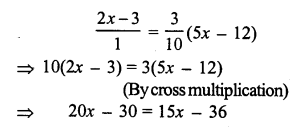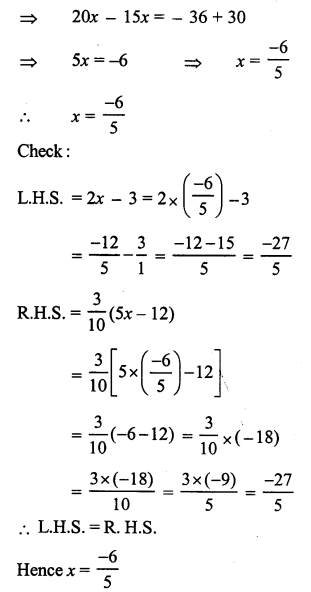Question 18.
Solution: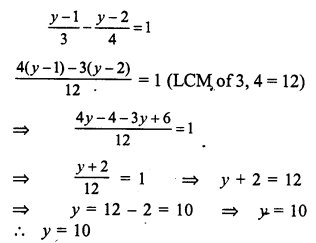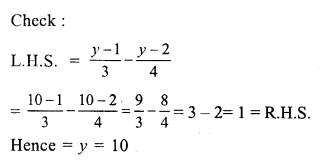Question 19.
Solution: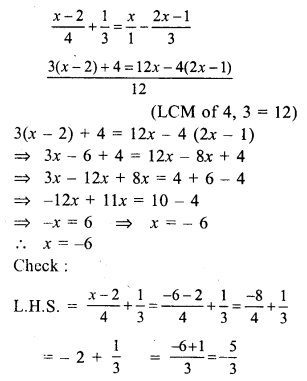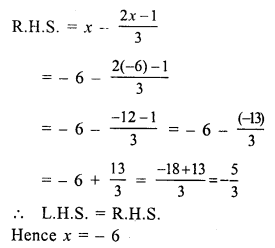Question 20.
Solution:Question 21.
Solution: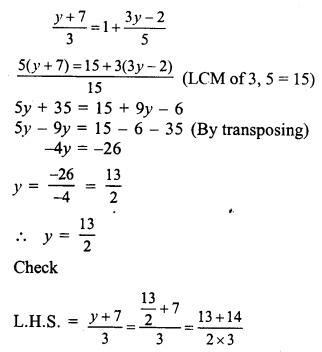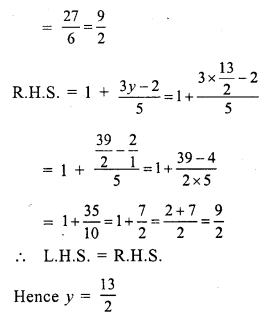Question 22.
Solution:Question 23.
Solution: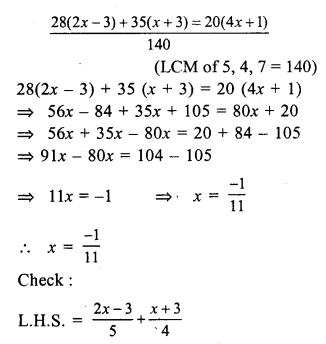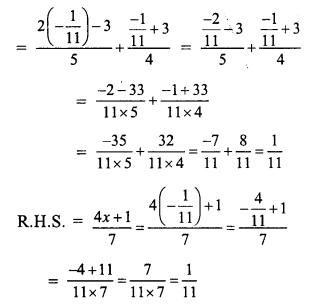Question 24.
Solution: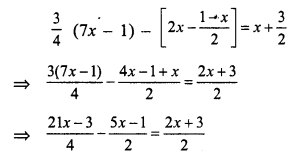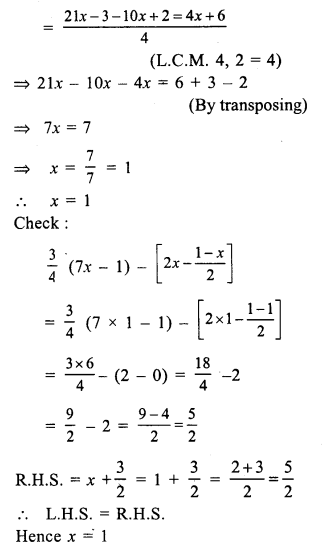Question 25.
Solution: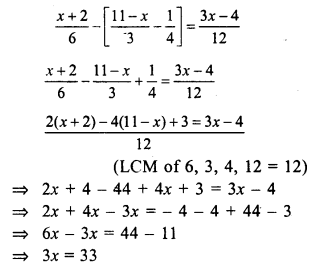Question 26.
Solution: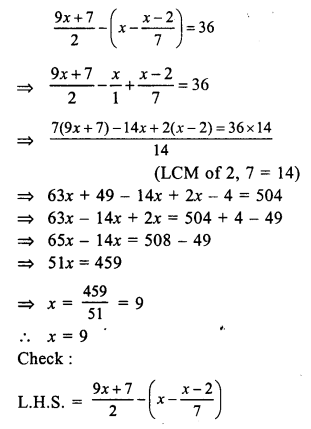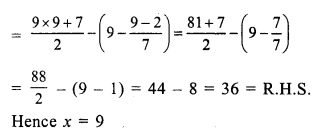Question 27.
Solution:Question 28.
Solution:
0.18 (5x – 4) = 0.5x + 0.8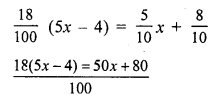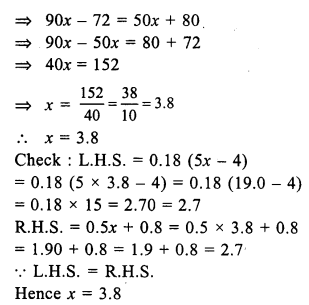Question 29.
Solution:
2.4 (3 – x) – 0.6 (2x – 3) = 0
⇒ 7.2 – 2.4x – 1.2x + 1.8 = 0
⇒ -2.4x – 1.2x = – (7.2 + 1.8).
L.H.S. = 2.4 (3 – x) – 0.6 (2x – 3)
⇒ 2.4 (3 – 2.5) – 0.6 (2 x 2.5 – 3)
⇒ 2.4 (0.5) – 0.6 (5 – 3)
⇒ 1.2 – 0.6 x 2 = 1.2 – 1.2 = 0 = R.H.S.
Hence x = 2.5

Question 30.
Solution:
0.5x – (0.8 – 0.2x) = 0.2 – 0.3x
⇒ 0.5x – 0.8 + 0.2x = 0.2 – 0.3x
⇒ 0.5x + 0.2x + 0.3x = 0.2 + 0.8
⇒ 1.0x = 1.0
⇒ x = 1
Check :
L.H.S. = 0.5x – (0.8 – 0.2x)
= 0.5 x 1 – (0.8 – 0.2 x 1)
= 0.5 – (0.8 – 0.2) = 0.5 – 0.6 = -0.1
R.H.S. = 0.2 – 0.3x = 0.2 – 0.3 x 1 = 0.2 – 0.3 = -0.1
L.H.S. = R.H.S.
Hence x = 1

Question 31.
Solution:Question 32.
Solution:Hope given RS Aggarwal Solutions Class 7 Chapter 7 Linear Equations in One Variable Ex 7A are helpful to complete your math homework.

If you have any doubts, please comment below. Learn Insta try to provide online math tutoring for you.